Courses

# Test : CSE Past Year Paper 2018

## 65 Questions MCQ Test GATE Computer Science Engineering(CSE) 2022 Mock Test Series | Test : CSE Past Year Paper 2018

Description
This mock test of Test : CSE Past Year Paper 2018 for GATE helps you for every GATE entrance exam. This contains 65 Multiple Choice Questions for GATE Test : CSE Past Year Paper 2018 (mcq) to study with solutions a complete question bank. The solved questions answers in this Test : CSE Past Year Paper 2018 quiz give you a good mix of easy questions and tough questions. GATE students definitely take this Test : CSE Past Year Paper 2018 exercise for a better result in the exam. You can find other Test : CSE Past Year Paper 2018 extra questions, long questions & short questions for GATE on EduRev as well by searching above.
QUESTION: 1

### What is the missing number in the following sequence? 2, 12, 60, 240, 720, 1440, .... 0

Solution:

The series starts with 2. We multiply first term with 6 to get second term 12 Then we multiply second term with 5 to get third term 60 Then we multiply third term with 4 to get fourth term 340 Then we multiply fourth term with 3 to get fifth term 720 Then we multiply fifth term with 2 to get fifth term 1440. Then we need to multiply with 1 and we get 1440 again.

QUESTION: 2

### What would be the smallest natural number which when divided either by 20 or by 42 or by 76 leaves a remainder ‘7’ in each case is_

Solution:

We need a number that can be written as 20x + 7, 42y + 7, 76z + 7 for three integers x, y and z. We basically need to find least common multiple of 20 (2 * 2 *5), 42(2 * 3 * 7) and 76(2 * 2 * 19) which is 2 * 2 * 5 * 3 * 7 * 19 = 20 * 21 * 19 = 420 * 19 = 7980 So our number is 7980 + 7 = 7987.
Note - You can also verify options using virtual calculator.

QUESTION: 3

### "From where are they bringing their books? _________ bringing _________ books from _________." The words that best fill the blanks in the above sentence are

Solution:

"From where are they bringing their books? Who bringing Whose books from Where." Who - A group as subject = They are Whose - of that group = their Where - from a place = there "From where are they bringing their books? They are bringing their books from there." So, option (B) is correct choice.

QUESTION: 4

A __________ investigation can sometimes yield new facts, but typically organized once are more successful.

Solution:

Meandering (as adjective) = proceeding in a convoluted or undirected fashion. Timely (as adjective) = done or occurring at a favourable or useful time; opportune. Consistent (as adjective) = acting or done in the same way over time, especially so as to be fair or accurate. Systematic (as adjective) = done or acting according to a fixed plan or system; methodical. Therefore, meandering is correct choice, all other options are similar to the word organized.

QUESTION: 5

The area of a square is ‘d’. What is the area of the circle which has the diagonal of the square as its diameter?

Solution: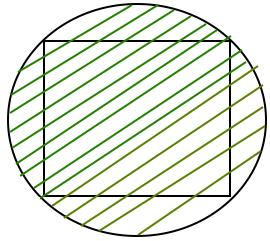One important observation to solve the question :
Diagonal of Square = Diameter of Circle.
Let side of square be x.
From Pythogorous theorem. Diagonal = √(2*x*x)
We know area of square = x * x = d
Diameter = Diagonal = √(2*d)
Area of Circle = π * √(d/2) * √(d/2) = 1/2 * π * d

QUESTION: 6

In the figure below, ∠DEC+∠BFC is equal to _______ .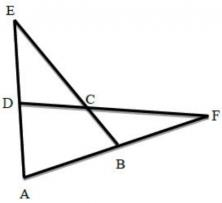Solution: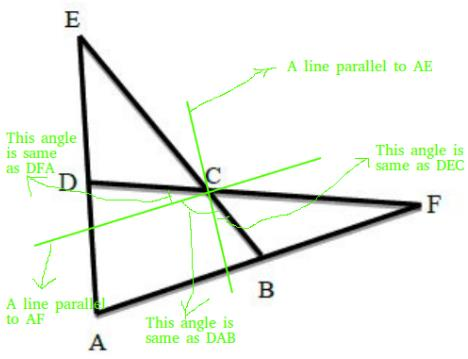QUESTION: 7

In a party, 60% of the invited guests are male and 40% are female. If 80% of the invited guests attended the party and if all the invited female guests attended, what would be the ratio of males to females among the attendees in the party?

Solution:

Let total males and females be 60x and 40x respectively.
Total number of people = (60x + 40x)
Total number of people who attended : 0.8(60x + 40x) = 80x
Let y males attended. It is given all 1females attended
40x + y = 80x
y = 40x which is same as females.
Alternative Approach - Lets total number of people = 100. Therefore, 60 are male and and 40 are female. But total 80 guests are attended and all 40 female attended the party. So, there remaining (80 - 40 = 40) attendees should be male. Then the ration of male to female among attendees is 40 : 40 = 1 : 1

QUESTION: 8

A six sided unbiased die with four green faces and two red faces is rolled seven times. Which of the following combinations is the most likely outcome of the experiment?

Solution:

Considering uniformly distributed outcomes, we get 4 greens and 2 reds in six throws. Then in one more throw, green is more likely. So, option (C) is correct.

QUESTION: 9

If pqr ≠ 0 and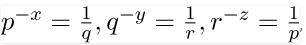what is the value of the product xyz ?

Solution:

Taking logs of given three values, we get
1/q = p-x -------(1)
1/r = q-y -------(2)
1/p = r-z -------(3)
1/q = p-x
= r-xz [Putting value of p from (3)]
= q-xyz [Putting value of r from (2)]
= 1 / qxyz
On comparing power of q both sides, we get xyz = 1
So, option (C) is correct.

QUESTION: 10

In appreciative of social improvement completed in a town, a wealthy philanthropist decided to give gift of Rs. 750 to each male senior citizen and Rs. 1000 for female senior citizens. There are total 300 senior citizens and th 8/9th of total men and 2/3rd of total women claimed the gift. What is amount of money philanthropist paid?

Solution:

Let there be x total men.
Total amount paid = x * 750 * 8/9 + (300 - x)*1000*2/3
= x*2000/3 + 300*1000*2/3 - x*2000/3
= 200000

QUESTION: 11

A queue is implemented using a non-circular singly linked list. The queue has a head pointer and a tail pointer, as shown in the figure. Let n denote the number of nodes in the queue. Let 'enqueue' be implemented by inserting a new node at the head, and 'dequeue' be implemented by deletion of a node from the tail.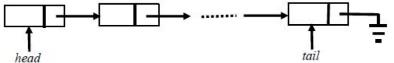Which one of the following is the time complexity of the most time-efficient implementation of 'enqueue' and 'dequeue, respectively, for this data structure?

Solution:

For Enqueue operation, performs in constant amount of time (i.e., Θ(1)), because it modifies only two pointers, i.e.,
Create a Node P.
P-->Data = Data
For Dequeue operation, we need address of second last node of single linked list to make NULL of its next pointer. Since we can not access its previous node in singly linked list, so need to traverse entire linked list to get second last node of linked list, i.e.,
While( temp-Next-->Next != NULL){
temp = temp-Next;
}
temp-->next = NULL;
Tail = temp;
Since, we are traversing entire linked for each Dequeue, so time complexity will be Θ(n). Option (B) is correct.

QUESTION: 12

The chromatic number of the following graph is _________ . Note - This was Numerical Type question.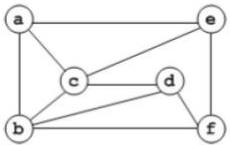Solution:

Chromatic number of given graph is 3.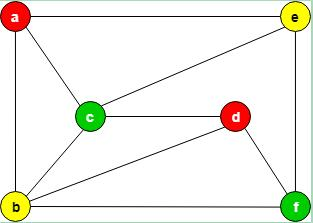Note that graph is Planar so Chromatic number should be less than or equal to 4 and can not be less than 3 because of odd length cycle. In other words if a graph is planar and has odd length cycle then Chromatic number can be either 3 or 4 only.

QUESTION: 13

Consider a matrix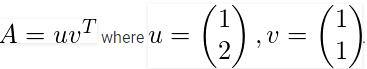Note that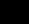denotes the transpose of v. The largest eigenvalue of A is ________ . Note -This was Numerical Type question.

Solution: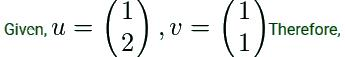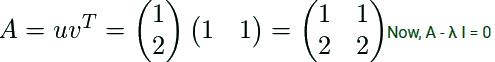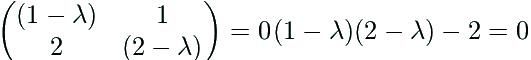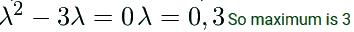QUESTION: 14

Consider the following C program:
#include <stdio.h>
int counter = 0;
int calc(int a, int b) {
int c;
counter++;
if (b == 3)
return (a * a * a);
else {
c = calc(a, b / 3);
return (c * c * c);
}
}
int main() {
calc(4, 81);
printf("%d", counter);
}

The output of this program is ________ . Note - This was Numerical Type question.

Solution: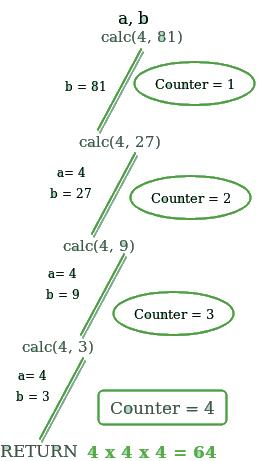QUESTION: 15

A 32 - bit wide main memory unit with a capacity of 1 GB is built using 256M X 4-bit DRAM chips. The number of rows of memory cells in the DRAM chip is 214. The time taken to perform one refresh operation is 50 nanoseconds. The refresh period is 2 milliseconds. The percentage (rounded to the closet integer) of the time available for performing the memory read/write operations in the main memory unit is _______ .
Note - This was Numerical Type question.

Solution:

Given, total number of rows is 214 and time taken to perform one refresh operation is 50 nanoseconds. So, total time taken to perform refresh operation = 214*50 nanoseconds = 819200 nanoseconds = 0.819200 milliseconds. But refresh period is 2 milliseconds. So, time spent in refresh period in percentage = (0.819200 milliseconds) / (2 milliseconds) = 0.4096 = 40.96% Hence, time spent in read/write operation = 100% - 40.96% = 59.04% = 59 (in percentage and rounded to the closet integer). So, answer is 59.

QUESTION: 16

Let G be a finite group on 84 elements. The size of a largest possible proper subgroup of G is _______ .
Note - This was Numerical Type question.

Solution:

According to Lagrange's theorem, states that for any finite group G, the order (number of elements) of every subgroup H of G divides the order of G. Therefore, possible subgroups of group on 84 elements are : 1, 2, 3, 4, 6, 7, 12, 14, 21, 28, 42, 84. Subgroups with element 1 and 84 are trivial groups. The size of a largest possible proper subgroup of G is 42.

QUESTION: 17

Consider a process executing on an operating system that uses demand paging. The average time for a memory access in the system is M units if the corresponding memory page is available in memory, and D units if the memory access causes a page fault. It has been experimental measured that the average time taken for a memory access in the process is X units. Which one of the following is the correct expression for the page fault rate experienced by the process?

Solution:

Given, average time for a memory access = M units if page hits, and average time for a memory access = D units if page fault occurred. And total/experimental average time taken for a memory access = X units. Let page fault rate is p. Therefore, Average memory access time = ( 1 - page fault rate) * memory access time when no page fault + Page fault rate * Memory access time when page fault → X = (1 - p)*M + p*D = M - M*p + p*D → X = M + p(D - M) → (X - M) = p(D - M) → p = (X - M) / (D - M) So, option (B) is correct.

QUESTION: 18

Which one the following is a closed form expression for the generating function of the sequence {an}, where an = 2n + 3 for all n = 0, 1, 2,...?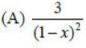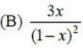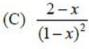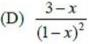Solution:

Given an = 2n + 3 Generating function G(x) for the sequence an is G(x) =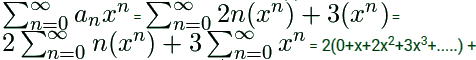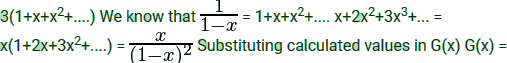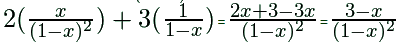QUESTION: 19

Which one of the following statements is FALSE?

Solution:

Type checking is done at semantic analysis phase and parsing is done at syntax analysis phase. And we know Syntax analysis phase comes before semantic analysis. So Option (B) is False. All other options seems Correct.

QUESTION: 20

The postorder traversal of a binary tree is 8, 9, 6, 7, 4, 5, 2, 3, 1. The inorder traversal of the same tree is 8, 6, 9, 4, 7, 2, 5, 1, 3. The height of a tree is the length of the longest path from the root to any leaf. The height of the binary tree above is ________ .
Note -This was Numerical Type question.

Solution:

Given, post-order - 8, 9, 6, 7, 4, 5, 2, 3, 1 and in-order - 8, 6, 9, 4, 7, 2, 5, 1, 3 Construct a binary tree from postorder and inorder traversal :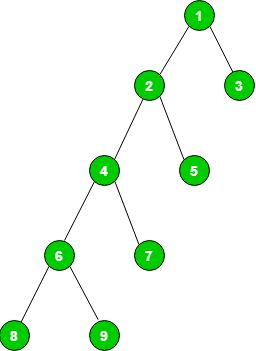The height of the binary tree above is 4.

QUESTION: 21

Let ⊕ and ⊙ denote the Exclusive OR and Exclusive NOR operations, respectively. Which one of the following is NOT CORRECT?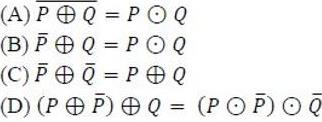Solution:

(A) (p⊕q)' = (pq' + p'q)' = (p'+q).(p+q') = (pp' +p'q' + qp + qq') = pq + p'q' = (p⊙q) (B) (p')⊕q = (p')q' + (p')'q = pq + p'q' = (p⊙q) (C) (p'⊕q') = (p')'.(q') + (p').(q')' = p.(q') + (p').q = p⊕q. (D) (p⊕(p'))⊕q =(pp''+ p'p')⊕q =(p+p')⊕q = 1⊕q = 1.q' + 0.q = q' And (p⊙(p'))⊙(q') = (pp' + p'.p'')⊙(q') = 0⊙(q') = 0(q') + 1.(q')' = q Please note that (p⊕q) = (p'⊙q) = (p⊙q') = (p'⊙q')' but not (p'⊙q'). Similarly, (p⊙q) = (p'⊕q) = (p⊕q') = (p'⊕q')' but not (p'⊕q'). So, option (D) is false.

QUESTION: 22

Consider the following statements regarding the slow start phase of the TCP congestion control algorithm. Note that cwnd stands for the TCP congestion window and MSS denotes the Maximum Segment Size.

1. The cwnd increase by 2 MSS on every successful acknowledgement.
2. The cwnd approximately doubles on every successful acknowledgedment.
3. The cwnd increase by 1 MSS every round trip time.
4. The cwnd approximately doubles every round trip time.

Which one of the following is correct?

Solution:

Slow-start begins initially with a congestion window size (cwnd) of 1, 2, 4 or 10 MSS. The value of the Congestion Window will be increased by one with each acknowledgement (ACK) received, effectively doubling the window size each round-trip time (approximately exponential). The cwnd increase by 1 MSS on every successful acknowledgement. Therefore, only statement (iv) is correct. Option (C) is true.

QUESTION: 23

The following are some events that occur after a device controller issues an interrupt while process L is under execution. (P) The processor pushes the process status of L onto the control stack. (Q) The processor finishes the execution of the current instruction. (R) The processor executes the interrupt service routine. (S) The processor pops the process status of L from the control stack. (T) The processor loads the new PC value based on the interrupt. Which of the following is the correct order in the which the events above occur?

Solution: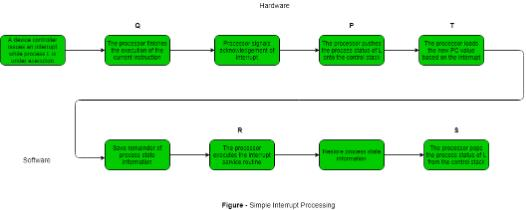QUESTION: 24

In an Entity-Relationship (ER) model, suppose R is a many-to-one relationship from entity set E1 to entity set E2. Assume that E1 and E2 participate totally in R and that the cardinality of E1 is greater that the cardinality of E2. Which one of the following is true about R?

Solution:

Since given relation is many to one :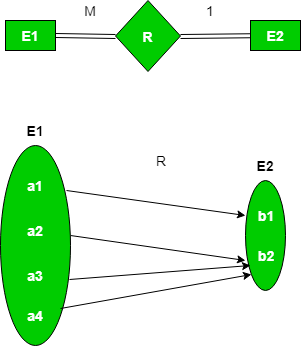Therefore, no entity in E1 can be related to more than one entity in E2 and an entity in E2 can be related to more than one entity in E1. Only option (A) is correct.

QUESTION: 25

The value of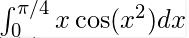correct to three decimal places (assuming that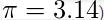is _______ .
Note -This was Numerical Type question.

Solution: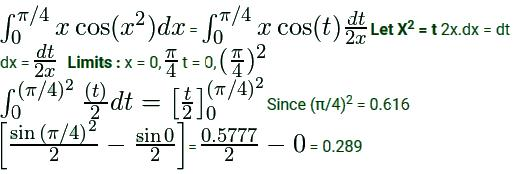QUESTION: 26

Let N be an NFA with n states. Let k be the number of states of a minimal DFA which is equivalent to N. Which one of the following is necessarily true?

Solution:

The minimum number of state in DFA will be 1 when we have only starting state and all other states will not be reachable from stating state. The minimum number of state in DFA will be 2n as the number of subsets of a set with n elements in the worst case. Therefore, k ≤ 2n Option (D) is correct. Related question - GATE | Gate IT 2008 | Question 6

QUESTION: 27

Two people, P and Q, decide to independently roll two identical dice, each with 6 faces, numbered 1 to 6. The person with the lower number wins, In case of a tie, they roll the dice repeatedly until there is no tie. Define a trial as a throw of the dice by P and Q. Assume that all 6 numbers on each dice are equi-probable and that all trials are independent. The probability (rounded to 3 decimal places) that one of them wins on the third trial is _______ .
Note - This was Numerical Type question.

Solution:

Given there are two identical dices, each having 6 faces. Favorable events for tie = {(1, 1), (2, 2), (3, 3), (4, 4), (5, 5), (6, 6)} Since two dice are thrown, total sample space will be 6 x 6 = 36 Therefore, P(tie) = 6/36 = 1/6 and Probability of not tie = (1 - 1/6) To one of them win on third trial, previous two trials should be tie. = 1/6 * 1/6 * (1 - 1/6) = 1/36 * 5/6 = 5/216 = 0.023 So, option (C) is correct.

QUESTION: 28

The set of all recursively enumerable languages is

Solution:

Recursive Enumerable Language are closed under Union, Intersection, Concatenation and Kleene Closure (but not Complementation). So, we can easily rule out option (A) and option (B) comes to be TRUE. Recursive Language are subset of REL, but option (C) is saying opposite, so Option (C) is FALSE. REL are countable, since set of all Turing Machines are countable. So option (D) is also FALSE. Only Option (B) is CORRECT.

QUESTION: 29

Consider the following two tables and four queries in SQL.
Book (isbn, bname), Stock (isbn, copies)

Query 1:
SELECT B.isbn, S.copies
FROM Book B INNER JOIN Stock S
ON B.isbn = S.isbn;

Query 2:
SELECT B.isbn, S.copies
FROM B B LEFT OUTER JOIN Stock S
ON B.isbn = S.isbn;

Query 3:
SELECT B.isbn, S.copies
FROM Book B RIGHT OUTER JOIN Stock S
ON B.isbn = S.isbn;

Query 4:
SELECT B.isbn, S.copies
FROM B B FULL OUTER JOIN Stock S
ON B.isbn = S.isbn;

Which one of the queries above is certain to have an output that is a superset of the outputs of the other three queries?

Solution:

In SQL the FULL OUTER JOIN combines the results of both left and right outer joins and returns all (matched or unmatched) rows from the tables on both sides of the join clause. So, option (D) is correct. See : Join (Inner, Left, Right and Full Joins), Inner Join vs Outer Join

QUESTION: 30

Consider a system with 3 processes that share 4 instances of the same resource type. Each process can request a maximum of K instances. Resource instances can be requested and released only one at a time. The largest value of K that will always avoid deadlock is _______ .
Note - This was Numerical Type question.

Solution:

Given, Number of processes (P) = 3 Number of resources (R) = 4 Since deadlock-free condition is:
R ≥ P(N − 1) + 1

Where R is total number of resources, P is the number of processes, and N is the max need for each resource.
4 ≥ 3(N − 1) + 1
3 ≥ 3(N − 1)
1 ≥ (N − 1)
N ≤ 2

Therefore, the largest value of K that will always avoid deadlock is 2. Option (B) is correct.

QUESTION: 31

Consider the sequential circuit shown in the figure, where both flip-flops used are positive edge-triggered D flip-flops.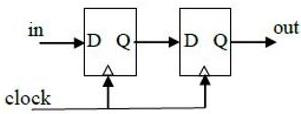The number of states in the state transition diagram of this circuit that have a transition back to the same state on some value of "in" is ______ .
Note - This was Numerical Type question.

Solution:

State Table :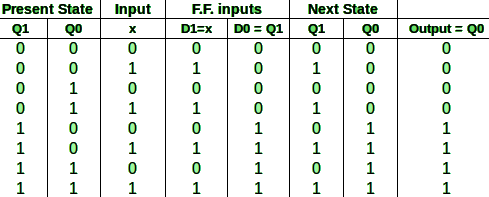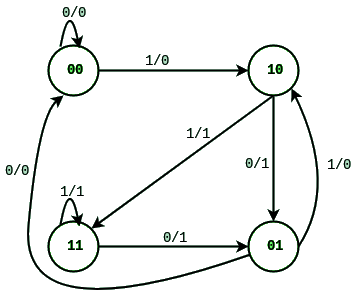Transition back to the same state means self loop, they are asking number of self loop states. Number of self loop states are 2 ( which are 00 and 11). Option (A) is CORRECT.

QUESTION: 32

Consider a long-lived TCP session with an end-to-end bandwidth of 1 Gbps (= 109 bits-per-second). The session starts with a sequence number of 1234. The minimum time (in seconds, rounded to the closest integer) before this sequence number can be used again is _______ .
Note - This was Numerical Type question.

Solution:

As sequence number field of TCP is 32 bits, so there are total 232 unique sequence number are possible (from 0 to 232-1), which is limit of TCP data. But if you want to send data more than 232 bytes in TCP, then you need to repeat this procedure after sending 232 bytes of data or unique sequence numbers. This concept is known as wrap around which allow sending unlimited data using TCP. Therefore, question is asking for wrap around time which is equal to pass all unique sequences first, i.e., 232, TCP assigns 1 sequence number to each byte of data.
Twrap−around = (Total data) / (Bandwidth)
= (232 bytes) / (109 bits per second)
= (232 * 8 bits) / (109 bits per second)
= 34.35 seconds = 34 (in seconds)
GATE' answer is same as either ceiling value or floor value (i.e., 34 and 35 both are correct). So, option (A) is correct.

QUESTION: 33

Consider the following C program.
#include <stdio.h>

struct Ournode {

char x, y, z;

};

int main() {

struct Ournode p = {'1', '0', 'a' + 2};

struct Ournode *q = &p;

printf("%c, %c", *((char *)q + 1), *((char *)q + 2));

return 0;

}

The output of this program is:

Solution:

'a' + 2 will be 'c', so Ournode p = {'1', '0', 'c'} and output will be 0, c. See: storage for Strings in C, string. Option (A) is correct.

QUESTION: 34

Match the following: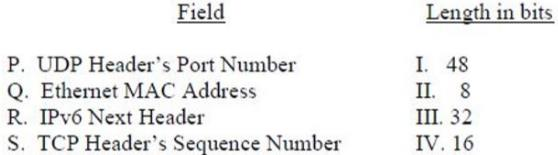Solution:

UDP Header's Port number is 16bits. Media Access Control (MAC) address is a globally unique identifier assigned to network devices, and therefore it is often referred to as hardware or physical address. MAC addresses are 6-byte (48-bits) in length and are written in MM:MM:MM:SS:SS:SS format. Next Header in IPv6 – Indicates either the first extension header (if present) or the protocol in the upper layer PDU (such as TCP, UDP, or ICMPv6). The size of this field is 8 bits. When indicating an upper layer protocol above the Internet layer, the same values used in the IPv4 Protocol field are used here. TCP Header's Sequence number (32 bits) - Has a dual role: If the SYN flag is set (1), then this is the initial sequence number. The sequence number of the actual first data byte and the acknowledged number in the corresponding ACK are then this sequence number plus 1. If the SYN flag is clear (0), then this is the accumulated sequence number of the first data byte of this segment for the current session. Therefore, option (C) P-IV, Q-I, R-II, S-III is correct.

QUESTION: 35

Consider the following processor design characteristics. I. Register-to-register arithmetic operations only II. Fixed-length instruction format III. Hardwired control unit Which of the characteristics above are used in the design of a RISC processor?

Solution:
• The RISC design philosophy generally incorporates a larger number of registers to prevent in large amounts of interactions with memory.
• The decision of RISC processor designers to provide simple addressing modes leads to uniform length instructions, so RISC instruction is of uniform fixed length.
• In the hardwired control unit, the control units use fixed logic circuits to interpret instructions and generate control signals from them. It is significantly faster than its counterpart but is rather inflexible. Most of the RISC processors are based on the hardwired control unit design approach.

So, all given characteristics are used in the design of a RISC processor. Option (D) is correct.

QUESTION: 36

Consider a storage disk with 4 platters (numbered as 0, 1, 2 and 3), 200 cylinders (numbered as 0, 1, … , 199), and 256 sectors per track (numbered as 0, 1, … 255). The following 6 disk requests of the form [sector number, cylinder number, platter number] are received by the disk controller at the same time: [120, 72, 2], [180, 134, 1], [60, 20, 0], [212, 86, 3], [56, 116, 2], [118, 16, 1] Currently head is positioned at sector number 100 of cylinder 80, and is moving towards higher cylinder numbers. The average power dissipation in moving the head over 100 cylinders is 20 milliwatts and for reversing the direction of the head movement once is 15 milliwatts. Power dissipation associated with rotational latency and switching of head between different platters is negligible. The total power consumption in milliwatts to satisfy all of the above disk requests using the Shortest Seek Time First disk scheduling algorithm is ______ .
Note -This was Numerical Type question.

Solution: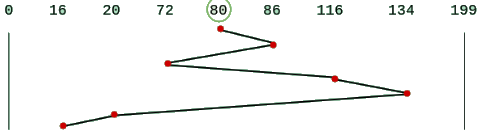Total Head movements in SSTF = (86-80) + (86-72) + (134-72) + (134-16) = 200 Power dissipated by 200 movements : P1 = 0.2 * 200 = 40 mW Power dissipated in reversing head direction once = 15 mW Number of time Head changes its direction = 3 Power dissipated in reversing head direction: P2 = 3 * 15 = 45 mW Total power consumption (in mW) is P1 + P2 = 40 mW + 45 mW = 85 mW So, answer is 85

QUESTION: 37

Consider an IP packet with a length of 4,500 bytes that includes a 20-byte IPv4 header ans 40-byte TCP header. The packet is forwarded to an IPv4 router that supports a Maximum Transmission Unit (MTU) of 600 bytes. Assume that the length of the IP header in all the outgoing fragments of this packet is 20 bytes. Assume that the fragmentation offset value stored in the first fragment is 0. The fragmentation offset value stored in the third fragment is ______ .
Note -This was Numerical Type question.

Solution:

MTU = 600 bytes and IP Header = 20 bytes So, Payload will be 600 - 20 = 580 bytes 580 is not multiple of 8, but we know fragment size should be multiple of 8. So fragment size = 576 bytes Kth fragmentation offset value = Fragment Size * (Kth fragment - 1) / Scaling Factor Offset value of 3rd fragment = 576 * (3 - 1) / 8 = 144

QUESTION: 38

Consider a simple communication system where multiple nodes are connected by a shared broadcast medium (like Ethernet or wireless). The nodes in the system use the following carrier-sense the medium access protocol. A node that receives a packet to transmit will carrier-sense the medium for 5 units of time. If the node does not detect any other transmission in this duration, it starts transmitting its packet in the next time unit. If the node detects another transmission, it waits until this other transmission finishes, and then begins to carrier-sense for 5 time units again. Once they start to transmit, nodes do not perform any collision detection and continue transmission even if a collision occurs. All transmissions last for 20 units of time. Assume that the transmission signal travels at the speed of 10 meters per unit time in the medium. Assume that the system has two nodes P and Q, located at a distance d meters from each other. P starts transmitting a packet at time t = 0 after successfully completing its carrier-sense phase. Node Q has a packet to transmit at time t = 0 and begins to carrier-sense the medium. The maximum distance d (in meters, rounded to the closest integer) that allows Q to successfully avoid a collision between its proposed transmission and P’s on going transmission is _______ .
Note -This was Numerical Type question.

Solution:

Node that receives the packet to transmit will carrier-sense the medium for 5 units. Any packet which arrives within 5 unit will be sensed and keep the channel busy. Given that Signal speed is 10 meter/time. Which means in 5 unit of time packet can travel 50 meters in max, that allow Q to successfully avoid the collision. So, option (A) is correct.

QUESTION: 39

A processor has 16 integer registers (R0, R1, … , R15) and 64 floating point registers (F0, F1, … , F63). It uses a 2-byte instruction format. There are four categories of instructions: Type-1, Type-2, Type-3, and Type 4. Type-1 category consists of four instructions, each with 3 integer register operands (3Rs). Type-2 category consists of eight instructions, each with 2 floating point register operands (2Fs). Type-3 category consists of fourteen instructions, each with one integer register operand and one floating point register operand (1R+1F). Type-4 category consists of N instructions, each with a floating point register operand (1F). The maximum value of N is _______ .
Note -This was Numerical Type question.

Solution:

Given, size of instruction format is 2 byte (= 16 bits), therefore number of instruction encoding = 216 Also, total number of bits in integer operand = log2(16 integer registers) = 4 Total number of bits in floating point operand = log2(64 floating point registers) = 6 So, number of encoding consumed: By type 1 instructions = 4×23×4 = 214 By type 2 instructions = 8×22×6 = 215 By type 3 instructions = 14×2(4+6) = 14336 Now, number of encoding left for type 4 instructions = 216 − (214 + 215 + 14336) = 2048 Therefore, total number of different instructions of type 4 instructions = 2048 /64 = 32 Please note that there is difference between number of different instructions and number of different encoding, a single instruction can have different encodings when the address part differs. So, answer is 32.

QUESTION: 40

Given a language L, define Li as follows:
L0 = {ε}
Li = Li-1∙L for all i>0
The order of a language L is defined as the smallest k such that Lk=Lk+1. Consider the language L1 (over alphabet 0) accepted by the following automaton.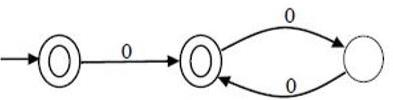The order of L1 is ______ . Note -This was Numerical Type question.

Solution:

L1 = ε + 0(00)* L0 = ε L1 = ε . (ε + 0(00)*)     = ε + 0(00)* = L1 L2 = L1 . L1 = L1 . L1     = (ε + 0(00)*) (ε + 0(00)*)     = ε + 0(00)* + 0(00)* + 0(00)* . 0(00)*     = ε + 0(00)* + 0(00)* 0(00)* = 0* Given automaton contains epsilon and even number of 0's and odd numbers of 0's. L3 = L2 . L1     = {0*} . {ε + 0(00)*} = 0* 0(00)* = 0* So, L2 = L3 = L2+1 So, smallest value of k = 2

QUESTION: 41

Consider the following solution to the producer-consumer synchronization problem. The shared buffer size is N. Three semaphores empty, full and mutex are defined with respective initial values of 0, N and 1. Semaphore empty denotes the number of available slots in the buffer, for the consumer to read from. Semaphore full denotes the number of available slots in the buffer, for the producer to write to. The placeholder variables, denoted by P, Q, R and S, in the code below can be assigned either empty or full. The valid semaphore operations are: wait() and sigmal().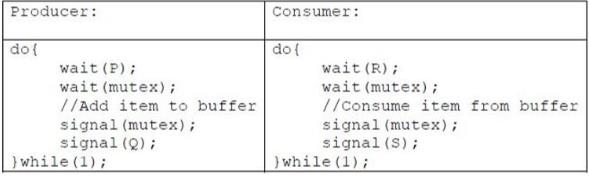Which one of the following assignments to P, Q, R and S will yield the correct solution?

Solution:

Given, Empty = 0 Full = N Mutex = 1 Since value of empty semaphore is 0, so you can not wait empty semaphore in first attempt.
Note - empty semaphore denotes the number of filled slots, so producer process must deal with empty and mutex semaphores. full semaphore denotes the number of empty slots so consumer process must deal with full and mutex semaphores. Option (A) causes starvation. OPtion (B) causes starvation. Option (D) since number of filled slots are 0 initially denoted by empty semaphore, so consumer process can not consume. So, this implimentation is wrong. Only P: empty, Q: full, R: full, S: empty is correct order to ensure deadlock-free and starvation free implementation. Option (C) is correct.

QUESTION: 42

Consider Guwahati (G) and Delhi (D) whose temperatures can be classified as high (H), medium (M) and low (L). Let P(HG) denote the probability that Guwahati has high temperature. Similarly, P(MG) and P(LG) denotes the probability of Guwahati having medium and low temperatures respectively. Similarly, we use P(HD), P(MD) and P(LD) for Delhi. The following table gives the conditional probabilities for Delhi’s temperature given Guwahati’s temperature.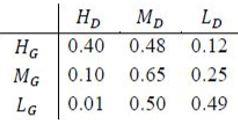Consider the first row in the table above. The first entry denotes that if Guwahati has high temperature (HG) then the probability of Delhi also having a high temperature (HD) is 0.40; i.e., P(HD ∣ HG) = 0.40. Similarly, the next two entries are P(MD ∣ HG) = 0.48 and P(LD ∣ HG) = 0.12. Similarly for the other rows. If it is known that P(HG) = 0.2, P(MG) = 0.5, and P(LG) = 0.3, then the probability (correct to two decimal places) that Guwahati has high temperature given that Delhi has high temperature is _______ .
Note -This was Numerical Type question.

Solution:

According to Bayes's Theorem: P(HD) = P(HD ∣ HG) × P(HG) + P(HD ∣ MG) × P(MG) + P(HD ∣ LG) × P(LG) P(HD) = 0.4 × 0.2 + 0.1 × 0.5 + 0.01 × 0.3 = 0.133 Therefore, P(HG ∣ HD) = P(HG ∩ HD) / P(HD) P(HG ∣ HD) = P(HD ∣ HG) × P(HG) / P(HD) P(HG ∣ HD) = 0.40 × 0.2 / P(HD) = 0.08 / 0.133 = 0.6015 So, option (A) is correct.

QUESTION: 43

Consider the following parse tree for the expression a#b$c$d#e#f, involving two binary operators $and #.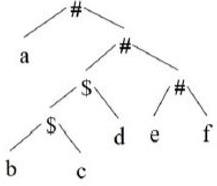Which one of the following is correct for the given parse tree? Solution: Since$ will be evaluated first, so has higher precedence with left associativity. Whereas # is right associative. In d#e#f, e#f will be evaluated first (refer given parse tree). Therefore, option (A) is Correct.

QUESTION: 44

Consider the following program written in pseudo-code. Assume that x and y are integers.

Count (x, y) {
if (y !=1 ) {
if (x !=1) {
print("*");
Count (x/2, y);
}
else {
y=y-1;
Count (1024, y);
}
}
}

The number of times that the print statement is executed by the call Count(1024, 1024) is _______ .
Note -This was Numerical Type question.

Solution:

Firstly, Count(1024, 1024) will be called and value of x will be deducted by x/2. 'x' will be printed 10 times. On count=10, value of x will become 1.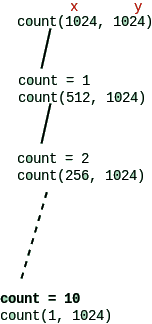Since x=1, inner else loop will start executing. On each call value of y will be decreased by 1 and will be executed until value of y becomes 1 (which is on 1023th call). Since count() is called recursively for every y=1023 and count() is called 10times for each y. Therefore, 1023 x 10 = 10230 Answer is 10230.

QUESTION: 45

The size of the physical address space of a processor is 2P bytes. The word length is 2W bytes. The capacity of cache memory is 2N bytes. The size of each cache block is 2M words. For a K-way set-associative cache memory, the length (in number of bits) of the tag field is

Solution:

Physical Address Space = 2P Bytes. Word Length is 2W bytes, which means each word is of size 2W bytes. Cache memory size = 2N Bytes and Tag Size = 2X Bytes. Physical address is P - W bits Number of blocks in cache = 2(N-W-M) It is a K-way set associative cache memory, each set in cache will have K-blocks. So, Number of sets = 2(N-W-M)/ K SET bits will be N-W-M-logk Offset bits will be M We know, TAG bits = Main memory bits - SET bits - offset bits So, TAG bits(x) = P - W - (N-M-W-logk)- M       = P - W - N + M + W + logk - M       x = P - N + logk Option (B) is Correct.

QUESTION: 46

Consider the following languages: I. {ambncpdq ∣ m + p = n + q, where m, n, p, q ≥ 0} II. {ambncpdq ∣ m = n and p = q, where m, n, p, q ≥ 0} III. {ambncpdq ∣ m = n = p and p ≠ q, where m, n, p, q ≥ 0} IV. {ambncpdq ∣ mn = p + q, where m, n, p, q ≥ 0} Which of the above languages are context-free?

Solution:

I. {ambncpdq ∣ m + p = n + q, where m, n, p, q ≥ 0}
m + p = n + q can also be written as m-n = q-p.
See the strings in given language : {ε ab, ad, bc, cd, abcd, abbc, aabb, aadd, acdd, bbcc, ccdd, aaabdd, aaabbd, bcccdd, aabcdd, .............} Given language is Context free, so Push Down Automata can be designed for this.   II. {ambncpdq ∣ m = n and p = q, where m, n, p, q ≥ 0}
m = n and p = q
See the strings in given language : { ε, ab, cd, abcd, aabbcd, abccdd, aaabbbccdd, ............} Definitely Context Free, hence PDA can be designed for this.   III. m=n=p and p ≠ q. Not Context free. IV. mn = p+q, Not Context free. Option (B) is Correct.

QUESTION: 47

Consider the following C code. Assume that unsigned long int type length is 64 bits.

unsigned long int fun(unsigned long int n) {

unsigned long int i, j = 0, sum = 0;

for( i = n; i > 1; i = i/2) j++;

for( ; j > 1; j = j/2) sum++;

return sum;

}
The value returned when we call fun with the input 240 is

Solution:

[sourcecode language=C] // n takes 2^40 unsigned long int fun(unsigned long int n) { // initialized sum = 0 unsigned long int i, j = 0, sum = 0; //First it takes i = n = 2^40, //then it divides i by 2 and incremented once j //each time, that's will make makes j = 40, for( i=n; i>1; i=i/2) j++; //Now the value of j = 40, //it divides j by 2 and incremented once sum //each time, that's will make makes sum = 5, for( ; j>1; j=j/2) sum++; //returns sum = 5 return sum; } [/sourcecode]

QUESTION: 48

Consider the minterm list form of a Boolean function F given below.
F(P, Q, R, S) = Σm(0, 2, 5, 7, 9, 11) + d(3, 8, 10, 12, 14)
Here, m denotes a minterm and d denotes a don't care term. The number of essential prime implicants of the function F is ______ .
Note: This was Numerical Type question.

Solution:

Essential Prime Implicants are those subcubes (groups) which cover atleast one minterm that can’t be covered by any other prime implicant. Essential prime implicants (EPI) are those prime implicants which always appear in final solution.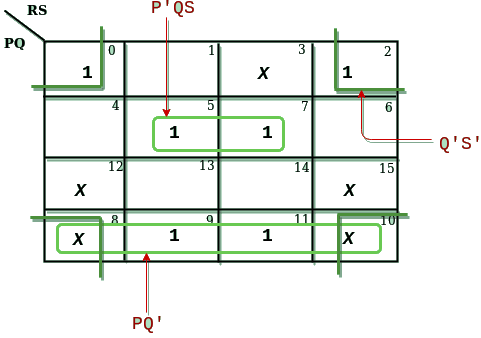There are three prime implicants P'QS, PQ' and Q'S'. Also, all of them are essential. Therefore, the number of essential prime implicants of function F is 3.

QUESTION: 49

Consider the following undirected graph G: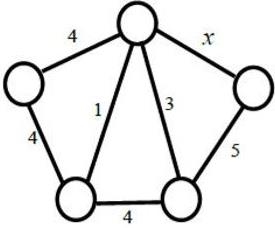Choose a value for x that will maximize the number of minimum weight spanning trees (MWSTs) of G. The number of MWSTs of G for this value of x is _________ .
Note -This was Numerical Type question.

Solution:

To maximize the number of minimum weight spanning trees of G, the value of x will be 5 because it will have two more choices for corner vertex which will maximize maximum number of MSTs. Now, according to kruskal algorithm for MST:

1. Edges with weights 1 and 3 will be selected first,
2. Now bottom edge with weight 4 will not be selected as will cause cycle on MST,
3. both corner vertices have two-two choices to select the vertices, so these corner edges with weights 4 and 5 will resultant 2*2 = 4 MSTs.

Therefore, total number of MSTs are 2*2 = 4, which is answer. Option (A) is correct.

QUESTION: 50

Consider the following problems. L(G) denotes the language generated by a grammar G. L(M) denotes the language accepted by a machine M. (I) For an unrestricted grammar G and a string w, whether wϵL(G) (II) Given a Turing machine M, whether L(M) is regular (III) Given two grammar G1 and G2, whether L(G1) = L(G2) (IV) Given an NFA N, whether there is a deterministic PDA P such that N and P accept the same language Which one of the following statement is correct?

Solution:

I, II and III are undecidable, refer this: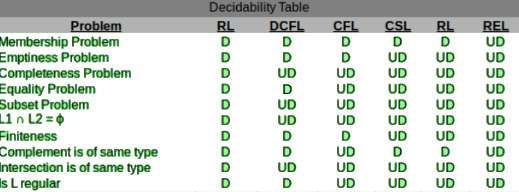Coming to IV, every regular language is Deterministic-CFL, which is a trivial property. Option (D) is Correct.

QUESTION: 51

Assume that multiplying a matrix G1 of dimension p×q with another matrix G2 of dimension q×r requires pqr scalar multiplications. Computing the product of n matrices G1G2G3 ..... Gn can be done by parenthesizing in different ways. Define GiGi+1 as an explicitly computed pair for a given paranthesization if they are directly multiplied. For example, in the matrix multiplication chain G1G2G3G4G5G6 using parenthesization (G1(G2G3))(G4(G5G6)), G2G3 and G5G6 are only explicitly computed pairs. Consider a matrix multiplication chain F1F2F3F4F5, where matrices F1,F2,F3,F4 and F5 are of dimensions 2×25,25×3,3×16,16×1 and 1×1000, respectively. In the parenthesization of F1F2F3F4F5 that minimizes the total number of scalar multiplications, the explicitly computed pairs is/are

Solution:

Matrix F5 is of dimension 1 X 1000, which is going to cause very much multiplication cost. So evaluating F5 at last is optimal. Total number of scalar multiplications are 48 + 75 + 50 + 2000 = 2173 and optimal parenthesis is ((F1(F2(F3 F4)))F5). As concluded, F3, F4 are explicitly computed pairs. Option (C) is Correct.

QUESTION: 52

Consider the following C program:

#include <stdio.h>

void fun1(char *s1, char *s2) {

char *temp;

temp = s1;

s1 = s2;

s2 = temp;

}

void fun2(char **s1, char **s2) {

char *temp;

temp = *s1;

*s1 = *s2;

*s2 = temp;

}

int main() {

char *str1 = "Yes", *str2 = "No";

fun1(str1, str2);

printf("%s %s", str1, str2);

fun2(&str1, &str2);

printf("%s %s", str1, str2);

return 0;

}
The output of the program above is

Solution:

fun1(char *s1, char *s2) Above function scope is local, so the value changed here won't affect actual parameters. SO the values will be 'Yes No'.   fun2(char **s1, char **s2) In this function value is pointer to pointer, so it changes pointer of the actual value. So values will be 'No Yes' Answer is 'Yes No No Yes'   Option (A) is correct.

QUESTION: 53

Consider the unsigned 8-bit fixed point binary number representation, below, b7b6b5b4b3 ⋅ b2b1b0 where the position of the binary point is between b3 and b2 . Assume b7 is the most significant bit. Some of the decimal numbers listed below cannot be represented exactly in the above representation: (i) 31.500 (ii) 0.875 (iii) 12.100 (iv) 3.001 Which one of the following statements is true?

Solution:

(i) 31.500 can be represented as : (31.5)10 = (11111.100)2
24 + 23 22 + 21 + 20 + 2-1 = 16 + 8 + 4 + 2 + 1 + 0.5 = (31.05)10

(ii) 0.875 can be represented as : (0.875)10 = (00000.111)2
2-1 + 2-2 2-3 = 0.50 + 0.25 + 0.125 = (0.875)10

(iii) and (iv) can not be be represented.   Therefore, option (C) is Correct.

QUESTION: 54

Consider the first-order logic sentence
φ ≡ ∃s∃t∃u∀v∀w∀x∀y ψ(s, t, u, v, w, x, y)
where ψ(s, t, u, v, w, x, y) is a quantifier-free first-order logic formula using only predicate symbols, and possibly equality, but no function symbols. Suppose φ has a model with a universe containing 7 elements. Which one of the following statements is necessarily true?

Solution:

Let's interpret the problem this way : ∀ are always True and ∃ are always False for empty sets. So there exists at least one model with universe of size 3 (or less than). Therefore, option (A) is necessarily TRUE.

QUESTION: 55

In a system, there are three types of resources: E, F and G. Four processes P0, P1, P2 and P3 execute concurrently. At the outset, the processes have declared their maximum resource requirements using a matrix named Max as given below. For example, Max[P2, F] is the maximum number of instances of F that P2 would require. The number of instances of the resources allocated to the various processes at any given state is given by a matrix named Allocation. Consider a state of the system with the Allocation matrix as shown below, and in which 3 instances of E and 3 instances of F are the only resources available.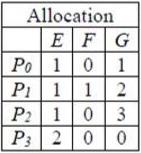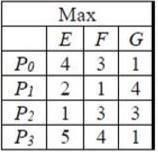From the perspective of deadlock avoidance, which one of the following is true?

Solution: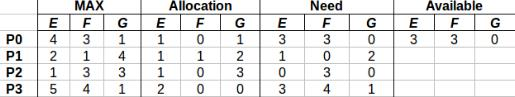Available (3, 3, 0), which can satisfy either P0 or P2. Take P0 <3, 3, 0>. After completion we have (3, 3, 0) + (1, 0, 1) = (4, 3, 1) Take P2 <0, 3, 0>. After completion we have (4, 3, 1) + (1, 0, 3) = (5, 3, 4) Take P1 <1, 0, 2>. After completion we have (5, 3, 4) + (1, 1, 2) = (6, 4, 6) Take P3 <3, 4, 1>. After completion we have (6, 4, 6) + (2, 0, 0) = (8, 4, 6) Safe sequence : P0-->P2-->P1-->P3 Therefore, option (A) is Correct.

QUESTION: 56

Let G be a graph with 100! vertices, with each vertex labelled by a distinct permutation of the numbers 1, 2, ..., 100. There is an edge between vertices u and v if and only if the label of u can be obtained by swapping two adjacent numbers in the label of v. Let y denote the degree of a vertex in G, and z denote the number of connected components in G. Then y + 10z = _______ . Note -This was Numerical Type question.

Solution:

There is an edge between vertices u and v iff the label of u can be obtained by swapping two adjacent numbers in the label of v. Then the set of swapping numbers will be {(1, 2), (2, 3), ...........(9, 9)} There will be 99 such sets, i.e. number of edges = 99 and each vertex will have 99 edges corresponding to it.
Say graph with 3! vertices, then vertices will be like {1, 2, 3}, {1, 3, 2}, {2, 1, 3}, {2, 3, 1}... Let's pick vertex {123}, degree will be 2 since it will be connected with two other vertices {213} and {132}. We can conclude that for n, degree will be n-1.
SO, degree of each vertex = 99 (as said y) As the vertices are connected together, the number of connected components formed will be 1 (as said z).
y+10z = 99+10(1) = 109

QUESTION: 57

The number of possible min-heaps containing each value from {1, 2, 3, 4, 5, 6, 7} exactly once is _______. Note -This was Numerical Type question.

Solution:

Set minimum element as root (i.e 1), now 6 are remaining and left subtree will have 3 elements, each left subtree combination can be permuted in 2! ways. Total ways to design min-heap with 7-elements =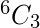*2! * 2! = 20*2*2 = 80 Alternative approach - Total number of min or max heap tree with 1 to N elements are using recurrence relation:
T(N) =(N-1)Ck * T(k) * T(N-k-1), where k = number of nodes on left subtree
T(1) = 1
T(2) = 1
T(3) = 2
T(4) = 3C2 * T(2) * T(1) = 3
T(5) = 4C3 * T(3) * T(1) = 8
T(6) = 5C3 * T(3) * T(2) = 20
T(7) = 5C3 * T(3) * T(3) = 80

QUESTION: 58

Let N be the set of natural numbers. Consider the following sets, P: Set of Rational numbers (positive and negative) Q: Set of functions from {0, 1} to N R: Set of functions from N to {0, 1} S: Set of finite subsets of N Which of the above sets are countable?

Solution:

Set of Rational numbers (+ve or -ve) are countable.. Set of functions from {0, 1} to N are countable because it has one to one correspondence to N. Set of functions from N to {0, 1} is uncountable, because it has one to one correspondence to set of real numbers between (0 and 1). Set of finite subsets of N is countable. Sets P, Q and S are countable, therefore option (D) is Correct.

QUESTION: 59

Consider the relations r(A, B) and s(B, C), where s.B is a primary key and r.B is a foreign key referencing s.B. Consider the query
Q: r⋈(σB<5(s))
Let LOJ denote the natural left outer-join operation. Assume that r and s contain no null values. Which one of the following is NOT equivalent to Q?

Solution:

Since, we are joining/LOJ using attribute B which is primary key of table s and foreign key of table r. So, we need to apply condition σB<5 on left table of join always, i.e., table r because left outer join (LOJ) returns all the values from an inner join plus all values in the left table that do not match to the right table. So, option (C) is correct.

QUESTION: 60

Consider the weights and values of items listed below. Note that there is only one unit of each item.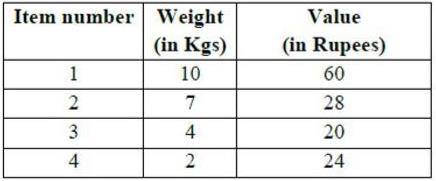The task is to pick a subset of these items such that their total weight is no more than 11 Kgs and their total value is maximized. Moreover, no item may be split. The total value of items picked by an optimal algorithm is denoted by Vopt. A greedy algorithm sorts the items by their value-to-weight ratios in descending order and packs them greedily, starting from the first item in the ordered list. The total value of items picked by the greedy algorithm is denoted by Vgreedy. The value of Vopt − Vgreedy is ______ .
Note -This was Numerical Type question.

Solution: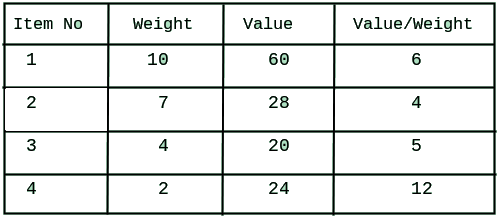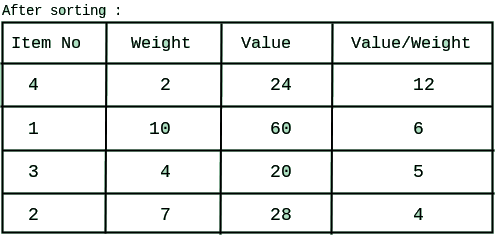First we will pick item_4 (Value weight ratio is highest). Second highest is item_1, but cannot be picked because of its weight. Now item_3 shall be picked. item_2 cannot be included because of its weight. Therefore, overall profit by Vgreedy = 20+24 = 44 Hence, Vopt - Vgreedy = 60-44 = 16 So, answer is 16.

QUESTION: 61

The instruction pipeline of a RISC processor has the following stages: Instruction Fetch (IF), Instruction Decode (ID), Operand Fetch (OF), Perform Operation (PO) and Writeback (WB), The IF, ID, OF and WB stages take 1 clock cycle each for every instruction. Consider a sequence of 100 instructions. In the PO stage, 40 instructions take 3 clock cycles each, 35 instructions take 2 clock cycles each, and the remaining 25 instructions take 1 clock cycle each. Assume that there are no data hazards and no control hazards. The number of clock cycles required for completion of execution of the sequence of instruction is ______ .
Note - This was Numerical Type question.

Solution:

Given, total number of instructions (n) = 100 Number of stages (k) = 5 Since, if n instructions take c cycle, so (c-1) stalls will occur for these instructions. Therefore, the number of clock cycles required = Total number of cycles required in general case + Extra cycles required (here, in PO stage) = (n + k - 1) + Extra cycles = (100 + 5 -1) + 40*(3-1)+35*(2-1)+20*(1-1) = (100 + 4) + 40*2+35*1+20*0 = 104 + 115 = 219 cycles So, option (A) is correct.

QUESTION: 62

Let G be a simple undirected graph. Let TD be a depth first search tree of G. Let TB be a breadth first search tree of G. Consider the following statements. (I) No edge of G is a cross edge with respect to TD. (A cross edge in G is between two nodes neither of which is an ancestor of the other in TD). (II) For every edge (u, v) of G, if u is at depth i and v is at depth j in TB, then ∣i − j∣ = 1. Which of the statements above must necessarily be true?

Solution:

There are four types of edges can yield in DFS. These are tree, forward, back, and cross edges. In undirected connected graph, forward and back egdes are the same thing. A cross edge in a graph is an edge that goes from a vertex v to another vertex u such that u is neither an ancestor nor descendant of v. Therefore, cross edge is not possible in undirected graph. So, statement (I) is correct. For statement (II) take counterexample of complete graph of three vertices, i.e., K3 with XYZ, where X is source and Y and Z are in same level. Also,there is an edge between vertices Y and Z, i.e., |i-j| = 0 ≠ 1 in BFS. So, statement became false. Option (A) is correct.

QUESTION: 63

Consider a matrix P whose only eigenvectors are the multiples of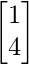. Consider the following statements. (I) P does not have an inverse (II) P has a repeated eigenvalue (III) P cannot be diagonalized Which one of the following options is correct?

Solution:

Repeated eigenvectors come from repeated eigenvalues. Therefore, statement (I) may not be correct, take any Identity matrix which has same eigenvalues but determinant so inverse exists. A matrix with repeated eigenvalues can or can not be diagonalized, repeated eigenvalues are necessary but not sufficient for a matrix to not be diagonalizable. But if all eigenvalues are distinct then we can be sure to be able to diagonalize it. In other words: as soon as all eigenvalues are distinct then we can be sure to be able to diagonalize it. Therefore, only statements (II) and (III) are necessarily true.

QUESTION: 64

Consider the following four relational schemas. For each schema, all non-trivial functional dependencies are listed, The underlined attributes are the respective primary keys.

• Schema I: Registration(rollno, courses) Field ‘courses’ is a set-valued attribute containing the set of courses a student has registered for. Non-trivial functional dependency rollno → courses
• Schema II: Registration (rollno, coursid, email) Non-trivial functional dependencies: rollno, courseid → email email → rollno
• Schema III: Registration (rollno, courseid, marks, grade) Non-trivial functional dependencies: rollno, courseid, → marks, grade marks → grade
• Schema IV: Registration (rollno, courseid, credit) Non-trivial functional dependencies: rollno, courseid → credit courseid → credit

Which one of the relational schemas above is in 3NF but not in BCNF?

Solution:
• Schema I: Registration(rollno, courses) Field ‘courses’ is a set-valued attribute containing the set of courses a student has registered for. Non-trivial functional dependency rollno → courses Since, rollno is primary key, so this relation is in BCNF as well as 3 NF.
• Schema II: Registration (rollno, coursid, email) Non-trivial functional dependencies: rollno, courseid → email email → rollno Since, {rollno, coursid} is primary key so rollno and coursid are prime attributes. email is non-prime attribute. Functional depedency (FD) rollno, courseid → email is in BCNF and 3NF, but FD email → rollno violates the rule of BCNF because email is not superkey. But it satifies rule of 3 NF because rollno is prime-attribute. So, overall this relation is in 3 NF but not in BCNF.
• Schema III: Registration (rollno, courseid, marks, grade) Non-trivial functional dependencies: rollno, courseid, → marks, grade marks → grade Since rollno, courseid is primary key, so rollno and courseid are prime attributes and marks and grade are non-prime attributes. FD rollno, courseid, → marks, grade satisfies BCNF as well as 3 NF. FD marks → grade does not satifies 3 NF because nither marks is superkey nor grade is prime-attribute. So, aslo can not be in BCNF. So, overall this relation is not in 3 NF and not in BCNF but it does not violates rule of 2 NF, so can be only in 2 NF.
• Schema IV: Registration (rollno, courseid, credit) Non-trivial functional dependencies: rollno, courseid → credit courseid → credit Since, rollno, courseid is primary key, so rollno and courseid are prime-attributes and credit is non-prime attribute. FD rollno, courseid → credit satifies BCNF as well as 3 NF. FD courseid → credit violates rule of 2 NF, so can not be in 2NF. So, overall this is not in 2 NF, 3 NF, and BCNF. But it is only in 1 NF.

Therefore only schema-II is in 3 NF but not in BCNF. Option (B) is correct.

QUESTION: 65

A lexical analyzer uses the following patterns to recognize three tokens T1, T2, and T3 over the alphabet {a,b,c}. T1: a?(b∣c)*a T2: b?(a∣c)*b T3: c?(b∣a)*c Note that ‘x?’ means 0 or 1 occurrence of the symbol x. Note also that the analyzer outputs the token that matches the longest possible prefix. If the string bbaacabc is processes by the analyzer, which one of the following is the sequence of tokens it outputs?

Solution:

0 or 1 occurrence of the symbol x.   T1 : (b+c)* a + a(b+c)* a T2 : (a+c)* b + b(a+c)* b T3 : (b+a)* c + c(b+a)* c Given String : bbaacabc Longest matching prefix is " bbaac " (Which can be generated by T3) The remaining part (after Prefix) "abc" (Can be generated by T3) So, the answer is T3T3